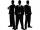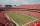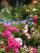TV commercials

For the typical one-hour prime-time television slot, the number of minutes of commercials is 3/8 of the minutes of the actual program. Determine how many minutes of the program are shown in that one hour.

Result

x =  43.6 min

Solution:Leave us a comment of this math problem and its solution (i.e. if it is still somewhat unclear...):Be the first to comment!To solve this verbal math problem are needed these knowledge from mathematics:

Need help calculate sum, simplify or multiply fractions? Try our fraction calculator. Do you have a linear equation or system of equations and looking for its solution? Or do you have quadratic equation?

Next similar math problems:

1. Jane plantsJane plants flowers in the garden. If she planted 12 every hour instead of 9 flowers, she would finish with the job an hour earlier. How many flowers does she plant?
2. Three menAlex is half younger than Jan, which is one-third younger than George. The sum of their ages is 48. How are these three men old?
3. The largerThe larger of two numbers is nine more than four times the smaller number. The sum of the two numbers is fifty-nine. Find the two numbers.
4. Two numbersThe sum of the two numbers is 1. Find both numbers if you know that half of the first is equal to one seventh of the second number.Number 118 divide into two addends, so first addend is 69 greater than 75% of the second addend.
6. FractionsThree-quarters of an unknown number are 4/5. What is 5/6 of this unknown number?
7. UN 1If we add to an unknown number his quarter, we get 210. Identify unknown number.
8. Unknown numberI think the number - its sixth is 3 smaller than its third.
9. Fraction + eqSolve following simple equation with fractions: -5/6(8+5b) = 75 + 5/3b
10. TreesAlong the road were planted 250 trees of two types. Cherry for 60 CZK apiece and apple 50 CZK apiece. The entire plantation cost 12,800 CZK. How many was cherries and apples?
11. TicketsOn the football tournament ticket cost 45 Kc for standing and 120kč for sitting. Sitting spectators was 1/3 more than standing. The organizers collected a total 12 300 Kc. How many seated and standing seats (spectators)?
12. Hotel roomsIn the 45 rooms, there were 169 guests, some rooms were three-bedrooms and some five-bedrooms. How many rooms were?
13. ATC campThe owner of the campsite offers 79 places in 22 cabins. How many of them are triple and quadruple?
14. Football seasonDalibor and Adam together scored 97 goals in the season. Adam scored 9 goals more than Dalibor. How many goals scored each?
15. Simple equation 6Solve equation with one variable: X/2+X/3+X/4=X+4
16. ClassroomThere are eighty more girls in the class than boys. Boys are 40 percent and girls are 60 percent. How many are boys and how many girls?
17. RosesOn the large rosary was a third white, half red, yellow quarter and six pink. How many roses was in the rosary?